## Chapter 6. Volumetric Geometry

This chapter covers advanced topics in volumetric geometry. For an understanding of basic volumetric terms and concepts, see “”. For an understanding of the API associated with these basic concepts, see “Read Brick Data from Disk”.

This chapter discusses the following advanced, volumetric topics:

## Data Structures

The Volumizer API defines several data structures to facilitate representation of volumetric geometry as well as the polygonal geometry that is derived to render the volumetric geometry, including voIndexedTetraSet and voIndexedFaceSet. Both classes are derived from the base class, voIndexedSet, and use voVertexData and voIndices internally.

### voVertexData

voVertexData is an array of records. Each record describes a set of properties of a vertex, such as:

• Vertex coordinates

• Vertex colors

• Vertex normals

• Texture coordinates

• Optional user-defined dataNote: The records are similar in structure and function to interleaved vertex arrays, as specified by the OpenGL 1.2 specification.

The number of properties per vertex is application-dependent. Vertex coordinates (three values) are always required; other properties are optional. The only supported value type is float.

To see the use of voVertexData in the context of an application, see “Allocate Storage for Bricks”.

#### Preferred Order of Values in Records

The API associates very little semantics with the per-vertex values. For example, all per-vertex properties are re-sampled and clipped during polygonize() action regardless of their interpretation. In rare cases, for example, voGeometryActions::draw(), the order of data actually matters. The preferred order of per-vertex data is:

1. User-defined

2. Normals

3. Voxel coordinates

4. Colors

5. Vertex coordinates

Any of the optional properties can be omitted. For example, if the application specifies only texture and vertex coordinates, each record consists of six values (s,t,r,x,y,z); the record type is T3F_V3F (Texture 3 floats, Vertex 3 floats).

The following commonly used combinations are supported:

 ```enum voInterleavedArrayType { DEFAULT, V3F, T3F_V3F, C3F_V3F, C4F_V3F, T3F_C3F_V3F, T3F_C4F_V3F, USER_V3F }; ```

USER_V3F is a catch-all type that can be used by applications that provide less common combinations of vertex properties. Applications that use USER_V3F or an unconventional order of properties should provide their own version of voGeometryActions::draw().

#### Creating an Instance of voVertexData

The constructor for voVertexData is

 ```voVertexData(int _countMax, int _valuesPerVertex, float* data=NULL); ```

You can construct an instance of voVertexData by requesting either:

• An empty instance of _countMax vertices, each with _valuesPerVertex values.

In this case, a new data area is allocated.

• Passing a pre-allocated array of floats to the constructor.

#### voVertexData Methods

In addition to a conventional set of constructor and accessors functions, voVertexData implements two methods:

• empty() sets the vertex count to zero, thereby marking the vertex array as empty.

• The array operator [] returns a pointer to the requested vertex record.

### voIndices

voIndices implements a simple array of indices, defined as follows:

 ```voIndices(int _countMax, int* indices=NULL); ```

voIndices is a component of several voIndexedSets. voIndices has no semantics associated with it.

In addition to a conventional set of constructor and accessors functions, voIndices implements the following two methods:

• empty() sets the index count to zero, thereby marking the object as empty.

• The array operator [] returns a requested element of the index array.

### voIndexedSet

voIndexedSet is an abstract class that contains one voVertexData and one voIndices and serves as a base class for both voIndexedTetraSet and voIndexedFaceSet. voIndexedSet has no semantics associated with its data.

In addition to a conventional set of constructor and accessors functions, voIndexedSet provides several methods that are shared by all the derived classes:

• empty() marks the given set as not containing any data.

• The array operator [] returns a pointer to an individual vertex record specified by the given index. (The semantics of such access varies among derived classes.)

#### voIndexedSetIterator

voIndexedSetIterator is an abstract class that enforces consistent traversal of voIndexedSets. For example, given a voIndexedFaceSet, that is, a set of polygons, you can use an instance of voIndexedFaceSetIterator to step through every vertex of each polygon.

In addition to a constructor, a voIndexedSetIterator has three methods:

• nextVertex() returns the next vertex of the current primitive, for example, a polygon, or NULL if all vertices of the current primitive were already traversed.

• nextPrimitive() steps to the next primitive.

• doneP() returns TRUE or FALSE depending on whether the whole indexed set was traversed.

### voIndexedFaceSet

voIndexedFaceSet describes a collection of indexed polygons. These polygons are often different, planar slices of the same volume, as shown in Figure 6-1.

Figure 6-1. Indexed Face Sets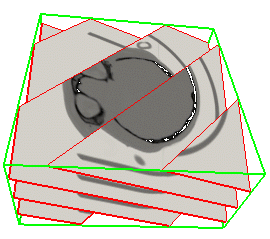The polygons are the product of voGeometryAction::polygonize(), in which the volume is sliced into polygons.

Each group of indices describes a single polygon.

#### Creating an Instance of voIndexedFaceSet

An empty instance of voIndexedFaceSet can be created by requesting a voIndexedFaceSet of _countVertices vertices, each with _valuesPerVertex fields, with, at most, _countIndices indices using the following constructor:

 ```voIndexedFaceSet (int _countMaxV, int _valuesPerVertex, int _countMaxI); ```

For example,

 ```voIndexedFaceSet *aFaceSet1 = voIndexedFaceSet(1000, 3, 5000); ```

This call constructs an empty instance of voIndexedFaceSet with a private vertex data array capable of holding 1,000 vertices each with three fields per vertex, and a private index array of 5,000 entries.

Another way to construct a face set is to specify a pre-allocated array of voVertexData and the maximum number of indices using the following constructor:

 ```voIndexedFaceSet (voVertexData* _vertexData, int _countMaxI); ```

For example:

 ```voVertexData *vertexData = voVertexData(1000, 3); voIndexedFaceSet *aFaceSet2 = voIndexedFaceSet(vertexData, 5000); ```

The advantage of supplying a pre-allocated array is that a single instance of voVertexData can be shared among many voIndexedFaceSets, for example:

 ```voVertexData *vertexData = voVertexData(1000, 3); voIndexedFaceSetPtr aFaceSetArray; for(int i1;j1<10;i1++) aFaceSetArray[i1] = voIndexedFaceSet(vertexData, 5000); ```

Pre-allotting the array reduces data fragmentation and lends itself more easily to parallelization.

#### Populating Face Sets with Polygons

All instances of voIndexedFaceSet constructed as above are initially empty. To populate them with polygons, use voIndexedFaceSet::appendPoly(), as follows:

 ```float vdata[] = { 100,100,100, 200,100,100, 200,200,100, }; aFaceSet->appendPoly(vdata, 3); ```

This code excerpt adds a triangle, defined by vdata[], to the face set.

If vdata in appendPoly() is NULL or points to the first empty record of the shared voVertexData array, Volumizer assumes that the application already placed the vertex data in the vertex array, so only indices are updated. Otherwise, the vertex data is copied from vdata into the vertex data array starting with the first available record. Appended vertex data are guaranteed to be copied into contiguous records.

#### voIndexedFaceSet Methods

voIndexedFaceSet inherits empty() from voIndexedSet. Calling empty() on an instance of voIndexedFaceSet constructed with a private vertex data area sets both the vertex and index counts to zero. The contents of the vertex data array and the index array is undefined after a call to empty().

Calling empty() on an instance constructed with a shared vertex data sets the index count to zero, but leaves the vertex data count unchanged. This is done in order to assure that other objects that may be sharing the vertex array are unaffected. Application should empty the vertex array explicitly in such situations:

 ```for(int i1;j1<10;i1++) aFaceSetArray[i1]->empty(); vertexData->empty(); ```

#### Deallocating Indexed Face Sets

Calling a destructor on an instance of voIndexedFaceSet deallocates all storage allocated by the constructor. That means that vertex data storage is deallocated only on instances created with the first version of the constructor. For instances that use a pre-allocated array, the application must deallocate the storage, for example:

 ```for(int i1;j1<10;i1++) delete aFaceSetArray[i1]; delete vertexData; ```

#### voIndexedFaceSetIterator

voIndexedFaceSetIterator facilitates traversal through a voIndexedfaceSet. For example, the following code steps though all polygons and prints out all the vertex coordinates for each polygon on a separate line:

 ```for(voIndexedFaceSetIterator iter(aFaceSet); iter.done();iter.nextPrimitive()) { while( (ptr = iter.nextVertex()) != NULL ) fprintf(stderr,”(%f %f %f) “, ptr[valuesPerVertex-3], ptr[valuesPerVertex-2], ptr[valuesPerVertex-1]); fprintf(stderr,”\n”); } ```

### voIndexedTetraSet

voIndexedTetraSet describes a collection of indexed tetrahedra; a collection of tetrahedra in Volumizer defines a solid shape. Each group of four indices in a tetraset form a single tetrahedron. The indices point into a vertex data array as in all other derivations of voIndexedSet.

#### Creating a voIndexedTetraSet

An empty instance of voIndexedTetraSet can be created by calling a constructor with countVertices, valuesPerVertex, and countIndex as arguments using the following constructor:

 ```voIndexedTetraSet(int _countMaxV, int _valuesPerVertex, int _countMaxI); voIndexedTetraSet(float* data, int _countMaxV, int _valuesPerVertex, int* _indices, int _indexMaxI); ```

_countMaxV is the number of vertices in the tetraset.

_valuesPerVertex are floating point values describing the vertices.

_countMaxI is the number of indices in the tetraset.

data must point to a linear array of floats that is at least _countMax*_valuesPerVertex long and the count is set to _countMax. If no data is specified, the storage is allocated and the vertex count is set to zero.

_indices points to index values.

_indexMaxI is the number of indices in the tetraset.

An alternative is to pass an array of floating point numbers describing the vertex data together with an array of indices using the following constructor:

 ```voIndexedTetraSet(voVertexData* data, voIndices* indices); ```

For example, the code below constructs an instance of voIndexedTetraSet describing a five-tetrahedron decomposition of a cube. Each vertex of the cube has color and coordinates associated with it.

 ``` const static float VSizeX = 128; const static float VSizeY = 128; const static float VSizeZ = 128; static float vtxData[8*6] = { 1, 0, 0, 0, 0, 0, 0, 1, 0, VSizeX, 0, 0, 0, 0, 1, VSizeX, VSizeY, 0, 0, 1, 1, 0, VSizeY, 0, 1, 0, 1, 0, 0, VSizeZ, 1, 1, 0, VSizeX, 0, VSizeZ, 1, 1, 1, VSizeX, VSizeY, VSizeZ, 0, 1, 1, 0, VSizeY, VSizeZ, }; static int cubeIndeces = { 0, 2, 5, 7, 3, 2, 0, 7, 1, 2, 5, 0, 2, 7, 6, 5, 5, 4, 0, 7, }; aTetraSet = new voIndexedTetraSet( vtxData, 8, 6, cubeIndeces, 20); ```

## Clipping Planes

Clipping planes into face sets is handled automatically either directly though OpenGL or with Volumizer API calls. In almost all cases, planes are clipped as part of voGeometryActions::polygonize() before drawing polygonized volumes.

In rare cases, an application might clip planes explicitly using voGeometryActions::clip(). For an example, see “Polygonizing Arbitrarily Oriented Cross Sections”.

## Arbitrarily Shaped Volumes of Interest

Decoupling appearance from geometry for volumetric shapes and using tetrahedral tessellations allows you to select VOIs bounded by arbitrarily complex constraints. For example, a spherical VOI can be crudely approximated with an icosahedron tessellated into tetrahedra. Including a conventional clip plane produces a semi-spherical VOI. It is important to notice, that this kind of modeling does not require any special programming, but merely a different set of geometry to be rendered. This way, the task of modeling is clearly decoupled from the rendering stage.

Similarly, one can render everything outside of a sphere by tessellating the space between two concentric spherical shells and making sure that the radius of the external sphere is large enough to encompass the whole volume. Rendering a volume minus a cylinder can be useful for looking at occluded objects. For example, in radiation therapy planning, it is common to visualize the prostate un-obscured by the hip bone and the bladder, but with enough anatomical context to facilitate treatment planning.

Figure 6-2 shows a tessellation that enables the mandible to be treated as a separate model part.

Figure 6-2. Arbitrarily Shaped VOI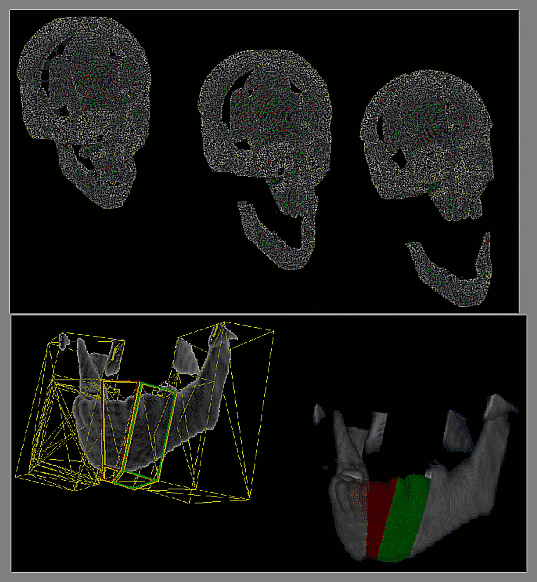## Using Higher-Level Geometric Primitives

The Volumizer API can render only those geometric shapes that are sets of tetrahedra. At times, however, it is more convenient to specify higher level geometric shapes, such as boxes or spheres. This section describes a set of helper routines that facilitate such high-level volumetric modeling.

The utility functions provide polyhedral shapes based on tetrahedra, pyramids, prisms, and hexahedra, as shown in Figure 2-11 on page 17. In addition, there are utilities that enable applications to easily model cylinders, cones, and spheres.

## Higher-Level Geometric Primitives and Solids

Volumizer offers the following higher-level polyhedral primitives:

• voutIndexedTetraFaceStrip

• voutIndexedTetraEdgeStrip

• voutIndexedHexaSet

• voutIndexedHexaStrip

• voutIndexedPyramidSet

• voutIndexedPyramidStrip

• voutIndexedPrismSet

• voutIndexedPrismFaceStrip

• voutIndexedPrismBaseStrip

• voutIndexedPrismEdgeStrip

There is also support for higher order solids (these are non-indexed):

• voutCone

• voutCylinder

• voutSphere

• voutGeoSphere

All these utility types are derived from an abstract base class, voutGeometry, and all the indexed types are also derived from voutIndexedSet. All of these classes provide a tessellate() method that returns a voIndexedTetraSet that is a tessellation of the given shape. For example:

 ```voutSphere *aSphere(0,0,0,100, 32, 32); voIndexedTetraSet *tetras = aSphere->tesselate(); ```

voutSphere implements a sphere abstract geometry class.

## Mixing Volumes and Surfaces

Rendering scenes containing conventional, surface-based and volumetric objects is essential for many applications. For example, for seismic data interpretation, it is often necessary to display oil wells and horizontal surfaces separating layers of geological material. Or, for the purpose of surgical simulation, you might like to render a scalpel, or a CAD model of a prosthetic device in the context of a patient's anatomy, as specified by a CT volume. Figure 6-3 was created by rendering a polygonal sphere inside of a CT volume.

Figure 6-3. Geometric (Sunglasses) and Volumetric Objects Rendered Together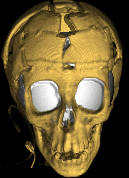The surface geometry being combined with volumes can be either opaque or translucent.

### Rendering Opaque Geometry with Volumes

1. Enable Z-buffering.

2. Render the surfaces in the scene.

3. Disable Z buffer writes (while leaving Z test enabled).

4. Render the three-dimensional volumes.

### Rendering Translucent or Overlapping Geometry with Volumes

The Volumizer API reduces all volumes to a set of semi-translucent polygons during the polygonization stage. To render translucent or overlapping geometry with volumes, you can use any renderer that knows how to handle transparency. You can merge the polygon lists from the volume and the surface models and render them in visibility order.

There are a number of techniques that can be used for that purpose. For example, Binary Space Partition (BSP) trees are commonly used to repeatedly depth sort a polygonal scene. Applications can take advantage of highly coherent structures of a volume-derived polygonal scene (many polygons share a supporting plane, and all planes are parallel) to create well balanced trees.

### Transient Geometry Caching During polygonize

The voIndexedFaceSets generated by polygonize() can often be reused. For example, for AXIS_ALIGNED sampling, the set of voIndexedFaceSets remains unchanged from view to view and can be cached without any consequences. Similarly, in VIEWPORT_ALIGNED mode, you can reuse sampling geometry from the previous frame if the two viewpoints are not very different. While such lazy evaluation may result in some quality degradation, it can be used for increased interactivity.

voGeometryActions::draw() initializes and maintains cache content. glwCache.cxx provides an example implementation of voGeometryActions::draw().

One thing to watch for when not using viewport-parallel sampling is perspective correction for your textures. When the sampling planes are parallel to the viewport, the textures do not need to be perspectively corrected because they are the same depth. In other cases, however, the underlying hardware correct the perspective otherwise artifacts may occur.

While two-dimensional textures are often perspective corrected, three-dimensional textures may not be (e.g., Impact) graphics in SGI's Indigo2 and Octane workstations). Therefore, it is safe to render in axis-aligned mode using two-dimensional interpolation, but it may not be safe to use other types of sampling with tree-dimensional textures.

## Rendering Multiple Volumes

Often you want to display images like these:

• Two non-associated, non-overlapping volumes, such as clouds.

• Two associated, overlapping volumes, such as a volume of radiation dose distribution and the corresponding CT data set describing the patient's anatomy, as shown in Figure 6-4.

• Two unassociated, overlapping volumes, such as a a CT and PET scan of a patient's head.

Figure 6-4. Overlapping Volumes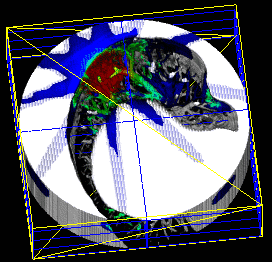Rendering non-associated, non-overlapping volumes is straightforward: the bricks of all the volumes in the scene are merged in a single list and sorted from back to front and the rest of the algorithm proceeds as described in Chapter 3, “Programming Template.”

There are, however, additional considerations when volumes overlap.

### Overlapping Volumes

When the volumes overlap, you must decide how to:

• Combine overlapping parts

• Overlap texture memory

#### Combining Overlapping Volumes

The semantics of the application often dictate how to combine overlapping volumes. For example, if the volumes are interpenetrating clouds, they need to absorb light according to the laws of optics.

If, on the other hand, a radiation dose distribution is to be “painted” over the anatomy, the values need to be merged through multiplicative blending, as shown in Figure 6-4. In this case, it may be necessary to use an offscreen buffer to produce individual slices.

#### Texture Memory Overlap

Every pair of bricks that overlaps has to be resident in the texture memory simultaneously. This condition halves the maximum brick size value and requires some of the bricks to be loaded more than once. Because texture downloading is expensive, you should create as few downloads as possible.

Figure 6-5. Merging Multiple Volumes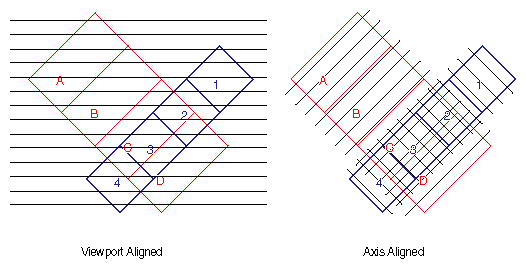Figure 6-5 shows multiple volumes merged under viewport aligned sampling (on the left) and under axis-aligned sampling (on the right). In this example, it's best if the bricks are small enough so that three bricks fit the texture memory simultaneously. Otherwise, the same brick may have to be reloaded several times.

#### Interpenetrating Polygons

If the sampling of two volumes by voGeometryActions::polygonize() does not occur along an identical set of surfaces, for example, in axis-aligned planes, the situation becomes complicated. In this scenario, the polygons resulting from the polygonization of each volume need to be depth sorted. The depth sorting is complicated by the fact that some of the polygons might be interpenetrating. To correctly depth sort the polygons, you need to use a BSP tree or some similar mechanism.

## Polygonizing Arbitrarily Oriented Cross Sections

Arbitrarily oriented cross sections, also called Multi-Planar Reformations (MPRs), of volumes are easily implemented on machines that support three-dimensional texture mapping hardware: the slicing plane is clipped, using voGeometryActions::clip(), to the volume's geometry and the brick boundaries and the resulting polygons are drawn with texturing enabled.

Note: The above technique may produce, what appears like geometric distortions on machines that do not perspectively correct three-dimensional textures (e.g., Impact graphics).

Without three-dimensional texture-mapping hardware, however, computing a tri-linearly filtered oblique slice through a volume is more challenging. This section describes how to accomplish this task using two-dimensional texture mapping and blending circuits commonly available on even the lowest-end machines.

Figure 6-6. MPR with Two-Dimensional Texture Mapping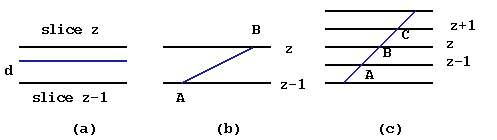Figure 6-6 shows a tri-linearly interpolated slice using two-dimensional texture mapping. The three instances in the figure show:

(a) A weighted average between two two-dimensional images

(b) An oblique slice between two two-dimensional images

(c) An oblique slice through a stack of two-dimensional images.

### Stack of Two-Dimensional Textures

Consider a stack of two-dimensional textures representing a three-dimensional volume of voxels. One can sample such volume only along any plane that coincides with any of the image planes to obtain a properly filtered image using only bi-linear interpolation.

Figure 6-6 shows a simple technique to sample along any plane that is parallel to the acquisition plane, but not necessarily coincident with any individual slice.

To obtain an image for the planes between the slices, use a weighted average of the two images, using the following procedure:

1. Enable the first two-dimensional texture.

2. Set the current color to (1-d, 1-d, 1-d, 1-d).

3. Draw the requested polygon.

4. Enable the second two-dimensional texture.

5. Set the current color to (d, d, d, d).

6. Enable additive blending before drawing the same polygon a second time.

7. Disable Z-testing or use the polygon offset has to assure that Z-fighting does not prevent the second pass from appearing the frame buffer.

8. Draw the second polygon.

### Using Angled Slices

The above procedure can be extended to obtain a properly-textured polygon that is cutting at an angle through two adjacent two-dimensional images, as shown in Figure 6-6(b).

The polygon is drawn twice using additive blending. However, rather than using constant opacity for the polygon, the opacity of each vertex is set to either 0 or 1, depending on whether the vertex falls into the active texture or not. For example, when texture Z is enabled, the opacity of vertex A is set to 0 and the opacity of vertex B is set to 1. Therefore, when the first texture is enabled, the pattern mapped onto the polygon is effectively multiplied by a linear ramp fading away in the parts of the polygon that are further away from the active image. Subsequently, the other texture is enabled, and the opacities of the vertices are reversed.

To minimize state changes once the texture is enabled, all polygons that use the same state should be drawn; for example, in Figure 6-6(c), once texture Z was enabled, polygons A-B and B-C should be drawn.

Figure 6-7 shows the partial results of the algorithm applied to every other slice.

Figure 6-7. MPR Bands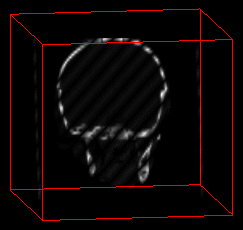You can compute an oblique slice through a stack of two-dimensional images by bounding each texture at most once and drawing the polygon exactly twice. The cross section, then, is computed at half the fill rate for two-dimensional textured polygons plus the overhead of computing the polygon's coordinates.

In the worst case, slicing through a n^3 volume requires computing 2 × n2 polygonal vertices. However, they can be easily found using tri-linear interpolation. This technique significantly reduces the overall complexity of tri-linearly sampling the entire slice.

### Multi-planar Reformatting Polygonization

polygonizeMPR() actions, described as follows, computes a set of polygons along an oblique section (Multi-planar Reformatting) clipped to brick boundaries. Two alternative actions differing by their inputs are provided. The first instance of the action will clip a plane, given its equation Ax+By+Cz+D=0. The second will clip an arbitrary polygon, described by their vertices.

 ```static int polygonizeMPR( float planeEquation, voBrickSet* aBrickSet, voIndexedFaceSet*** polygonSet, voInterleavedArrayType interleavedArrayFormat); static int polygonizeMPR( voVertexData* vData, voBrickSet* aBrickSet, voIndexedFaceSet*** polygonSet, voInterleavedArrayType interleavedArrayFormat); ```

The plane is given by its equation, planeEquation.

The input polygon is described by voVertexData.Note: Currently, this method is implemented only for two-dimensional textures.

Shading increases the realism of an image by applying lighting calculations to the object being rendered. For example, the image on the right in Figure 6-8 was shaded (using a Z-buffer shading technique), while the one on the left was rendered with simple opacity blending only.

Figure 6-8. Shading Off and On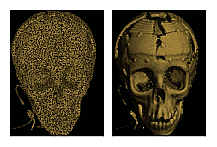In addition to looking more realistic, the shaded image enhances many surface details that are not apparent in the blended rendition, for example, the small bones in the nasal cavity, and staples holding the pieces of forehead together.

Currently, the API does not define how polygons produced by polygonize() are blended. Applications can use OpenGL's glBlendFunc() to select a suitable blending, for example, OVER or MIP.

A more sophisticated scenario calls for computation of per-voxel gradient. The gradient is used as an approximation for the surface normal in lighting calculations. You can implement gradient-based shading by maintaining a gradient volume in addition to the original voxel data.

A significantly more flexible technique that allows for on-the-fly per-voxel gradient computation is based on the tangent-space techniques that were recently proposed for bump mapping (consult SIGGRAPH97 Proceedings, and SIGGRAPH98 Course 17 by Tom McReynolds for details). In this multi-pass approach, each sampling polygon is drawn twice using blending operations to estimate the component of the gradient in the direction of the light source.

More specifically, the polygon is drawn first into a off-screen memory. Subsequently, the vertex coordinates of the polygon are offset by a unit in the direction of the light source (alternatively, the texture coordinates are shifted by a corresponding fraction in the opposite direction).

The offset polygon is drawn for the second time subtracting it from the first image (this operation can also be implemented efficiently with an accumulation buffer). Effectively, this operation computes the projection of the gradient vector onto the direction of the light vector, or the dot product of these two vectors. Once the subtracted image is computed it is copied into the frame buffer and blended using conventional OVER operator.

The main advantage of the technique is the ability to compute the shading directly from the intensity data thus avoiding memory bloat and bandwidth issues which plague algorithms that require storing of the pre-computed gradient volume. Further, it allows for post-lookup, post-interpolation gradient calculation that may be required by high quality applications. The main drawback is use of the un-normalized gradient, which can produce shading artifacts similar to using un-normalized vertex normals with conventional models.

A sample implementation of this technique is provided with the distribution.

## Volume Roaming

Many applications deal with very large volumes. In these cases, only a subregion of the volume can be displayed at any given time. This subregion is called the Volume of Interest (VOI).

The VOI can be of any volumetric shape. Figure 6-9 shows a cubical VOI in the midst of geological data.

Figure 6-9. Volume of Interest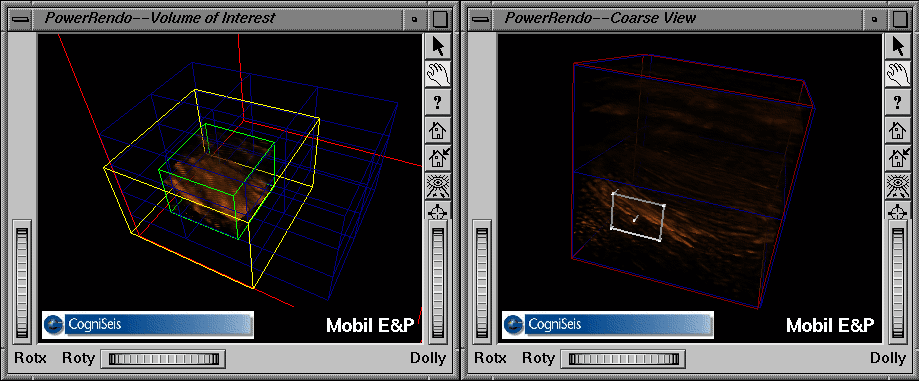### Implementing a Volume of Interest

You can enable a user to translate and scale a VOI, such as a cube or a sphere. For example, suppose you roam a 20483 (8 GB) volume bricked into 64 2563 bricks. The VOI is a 5123 cube, which can be translated and scaled. Every time the position or size of the VOI changes, the application has to:

1. Determine which bricks have shifted into the field of view.

2. Read those bricks in from the disk.

3. Determine which bricks have shifted out of the field of view.

4. Discard those bricks and mark them as inactive.

These tasks can be accomplished by defining the vertices of the geometry, voIndexedTetraSet, so they coincide with the vertices of the VOI rather than the vertices of the voxel data cube. The same approach can be used to manipulate arbitrarily shaped VOIs.

More importantly, thanks to virtualized (bricked) appearance, applications can control the amount of voxel data present in the memory and can implement just-in-time brick loading.

## Picking Volumetric Objects

Many applications are interested in selecting objects being drawn. For volumetric shapes such selection can be on two different levels: sub-part level and voxel level.

Applications that tessellate objects into individual parts may require that an individual part be selectable. For example, a mandible may have to be selected and moved around for maxillofacial surgery planning. For this type of per-part selection it is best to use OpenGL picking and selection mechanisms. Simply draw the faces of the tetrahedra defining the part just as if you were dealing with a conventional, surface-based model, using voGeometryActions::draw().

At the low level, an application may want to inspect individual voxels of a model. For example, a distance between two three-dimensional anatomical landmarks may be required for a morphometric measurement. Similarly, a seismic data interpretation application may want to automatically segment out a layer of geological material by simply pointing at it on the screen.

Voxel picking was provided for the purpose of analyzing the volume on a point by point basis. In order to make the picking functionality general purpose, the API does not make any application-specific decisions. Instead, an array of voxels along a line (typically the line of sight through the cursor) is returned. It is application's responsibility to do its own signal processing to extract features of interest, for example, find the closest voxel along the line of sight that has a value above the threshold.

To return an array of voxels and their coordinates for an intersected volumetric object, use the following voGeometryActions method:

 ```static voBool pick( int pixelPosition, int viewport, voIndexedTetraSet* tetraSet, voBrickSet* aBrickSet, voInterleavedArrayType interleavedArrayFormat, GLdouble modelMatrix, GLdouble projMatrix, float samplingPeriod, voBool flag, voVertexData* coordinates, void* voxels); ```

This method determines whether or not aBrickSet is intersected by a line-of-sight ray through the cursor. The method returns VO_TRUE if the brickset is intersected or VO_FALSE otherwise.

The equation of the line is determined from the (row, col) screen position, the viewport, and modelview/projection matrices. This line is intersected with the volume described by aTetraSet and aBrickSet. Sampling period determines how often to sample along this line. The routine computes the values of voxels and their respective coordinates.

The requested voxel data is returned in voxels in the format and voxel type of the brick set. If flag is set to VO_FALSE, the original voxel values along the line of sight are returned using nearest neighbor interpolation. If flag is set to VO_TRUE, the current graphics state is applied, for example, tri-linear interpolation, lookup tables, or interpolated-per-vertex colors.

The first voxel-coordinate pair refers to the intersection point on the volume closest to the viewpoint; the last voxel-coordinate pair refers to the intersection point on the volume furthest from the viewpoint. The coordinates along the line are returned in coordinates as specified by the voInterleavedArrayFormat enum, as defined in “Preferred Order of Values in Records”.

Setting flag to VI_TRUE, if it is implemented in hardware, is generally faster for a single-brick brick set, but may result in fully reloading each brick in a multi-brick brick set through the texture memory. Setting voxels to NULL returns vertex data but no voxel data so that the application can compute their own voxel values.

The other form of the same voGeometryActions method is:

 ```static voBool pick(int pixelPosition, int voewport, voIndexedTetraSet* tetraSet, voBrick* aBrick, voInterleavedArrayType interleavedArrayFormat, GLdouble modelMatrix, GLdouble projMatrix, float samplingPeriod, voBool flag, voVertexData* coordinates, void* voxels); ```

The second version of the method tests to see if a voBrick instead of a voBrickSet is intersected. Applications can use this overloaded method to apply hardware-assisted picking on a brick by brick basis. For example, the application can sort the bricks by depth and only apply the pick action to the closest brick thus reducing download requirements significantly.

## Auxiliary Methods

The following voGeometryActions auxiliary methods determine the position of the camera and the direction it is facing from OpenGL matrices, respectively:

 ```static void findCameraPosition( double eyePos, double viewDir, GLdouble modelMatrix, GLdouble projMatrix); static void findViewDirection ( double viewDir, GLdouble modelMatrix, GLdouble projMatrix); ```

findCameraPosition() determines the view direction and eye position given the modelview and projection matrices. If the projection matrix describes an orthographic projection, set viewDir to 0.0 and do not try to find the eye position. If the matrices were singular (for example, scaled by factor of 0.0 for projective shadows), voErrorNo is returned as BAD_VALUE and no eye position is found. The vector returned is in object space and points from the camera toward the volume.

findViewDirection() determines the viewing direction given the modelview and projection matrix. This should generally not be a problem unless the matrices are singular; for example, the view frustum is reduced to 0 depth by a scaling factor of 0.0. This is a common practice for the projective shadows technique. If the direction cannot be computed, voErrorNo is set to BAD_VALUE, and viewDir[] is set to (0.0,0.0,1.0). The vector returned is in object space and points from the volume toward the camera.